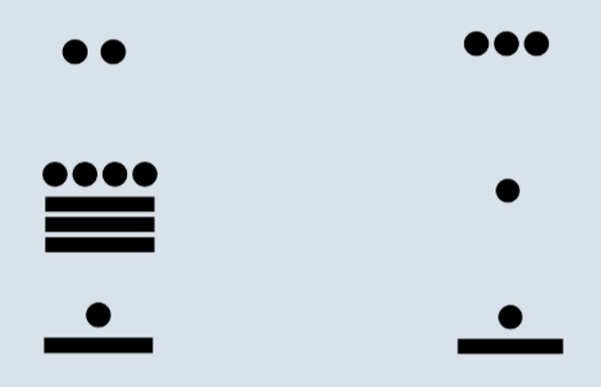# Positional number systems around the world

Positional number systems use the order or position of symbols to determine a number's value. For example the numbers 506 and 650 both use the same symbols, but the differing order of those symbols means they have different values.

## Mayan Numerals

The Mayan used a mixed base (20 and 360), positional number system. They used only three symbols, a snail shell representing 0, a dot representing 1, and a bar for 5:The numbers would be written vertically, with the bottom digit representing the 1s, the digit above would represent the 20s, and then instead of 400, the next digit represents the 360s. The sequence follows this pattern:

1, 20, 18x20, 18x202, 18x203, 18x204, ...

The deviation from a pure base 20 comes from the Mayan 360-day calendar.

The variation has the interesting result of some numbers not having unique representations. For example, 1,106 can be written in two ways:The first way is 6 + (19x20) + 2x360, and the second way is 6 + (1x20) + (3x360). The reason this happens is by exploiting the fact that there is a symbol for numbers up to 19 so the second digit can represent as high as 19x20, but the third digit can represent as low as 18x20.

Other examples of positional number systems include Babylonian numbers, Incan Quipa, and Rod Numerals.

## Rod Numerals

Rod numerals come from ancient East Asia over two thousand years ago. They use lines (or rods) to draw out each digit. Counting up to ten, first draw 4 vertical lines, then replace them with one horizontal line, and then add four more vertical lines.

Numbers are written left to right in base 10, with the rightmost digit being the 1s. For clarity, the direction digits are drawn alternates between starting with vertical lines and horizontal lines.